﻿ Effective Material Moduli for Composites

### Effective Material Moduli for Composites

Juraj Šarloši, Jozef Bocko

American Journal of Mechanical Engineering

## Effective Material Moduli for Composites

Juraj Šarloši1,, Jozef Bocko1

1Department of Applied Mechanics and Mechanical Engineering, Technical University in Košice, Košice, Slovakia

### Abstract

In this article we deal with deriving the elastic modulus of composite materials. Modulus values in each direction are various, for example in parallel direction and the perpendicular direction. It depends on the material properties for fibers from material for matrix, density of fibers in the composite material, as well as on whether it is a single or multi-layer composite material and from the direction the filaments in the matrix.

• Juraj Šarloši, Jozef Bocko. Effective Material Moduli for Composites. American Journal of Mechanical Engineering. Vol. 4, No. 7, 2016, pp 289-292. http://pubs.sciepub.com/ajme/4/7/11
• Šarloši, Juraj, and Jozef Bocko. "Effective Material Moduli for Composites." American Journal of Mechanical Engineering 4.7 (2016): 289-292.
• Šarloši, J. , & Bocko, J. (2016). Effective Material Moduli for Composites. American Journal of Mechanical Engineering, 4(7), 289-292.
• Šarloši, Juraj, and Jozef Bocko. "Effective Material Moduli for Composites." American Journal of Mechanical Engineering 4, no. 7 (2016): 289-292.

 Import into BibTeX Import into EndNote Import into RefMan Import into RefWorks

12
Prev Next

### 1. Introduction to Composite Material

Composite materials have at least two different material components which are bonded. The material response of a composite is determined by the material moduli of all constituents, the volume or mass fractions of the single constituents in the composite material, by the quality of their bonding, i.e. of the behavior of the interfaces, and by the arrangement and distribution of the fiber reinforcement, i.e. the fiber architecture.

The basic assumption made in material science approach models of fiber reinforced composites are:

• The bond between fibers and matrix is perfect

• The fibers are continuous and parallel aligned in each ply, they are packed regularly, i.e. the space between fibers uniform.

• Fiber and matrix materials are linear elastic, they follow approximately Hooke´s law and each elastic modulus is constant

• The composite is free of voids

Composite materials are heterogeneous, but in simplifying the analysis of composite structural elements in engineering applications, the heterogeneity of the material is neglected and approximately overlayed to a homogenous material. The most important composite in structural engineering applications are laminates and sandwiches. Each single layer of laminates or sandwich faces is in general a fiber reinforced lamina .

### 2. Elementary Mixture Rules for Fiber – Reinforced Laminate

One of the most important factor which determines the mechanical behavior of a composite material is the proportion of the matrix and the fibers expressed by their volume or their weight fraction. These fractions can be established for two phase composite in the following way. The volume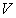of the composite is made from a matrix volume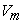and the fiber volume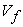. In a similar way the weight or mass fraction of fiber and matrices can be defined. The mass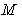of the composite is made from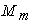and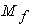. The rule of mixture is based on the statement that the composite property is the sum of the properties of each constituent multiplied by its volume fraction. For density of the composite material we can write :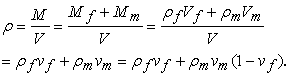(1)

In a actual lamina the fibre are randomly over the lamina cross-section and the lamina thickness is about 1mm and much higher than the fibre diameter (about 0,01mm).

### 3. Longitudial Moduls of Elasticity

When an unidirectional lamina is acted upon by either a tension or compression load parallel to the fibers, it can be assumed that the strains of the fibers, matrix and composite in the loading direction are the same.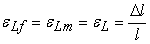(2)Download asVeiw figureFigures index
Figure 1. Mechanical model to calculate the effective Young´s modules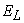The mechanical model has a parallel arrangement of fibres and matrix and the resultant axial force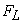of the composite is sheared by both fibre and matrix so that: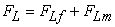or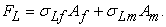(3)

With Hooke´s law it follows that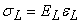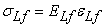(4)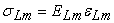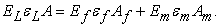(5)

If we divide with area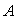we get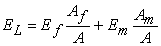(6)

with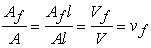(7)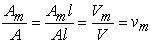(8)

and the effective moduluscan be written as follows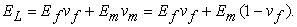(9)

The equation (9) is known as rule of mixture. The predicted values ofare in good agreement with experimental results. The stiffness in fiber direction is dominated by the fiber modulus. The ratio of the load taken by the fiber to the load taken by the composite is a measure of the load shared by the fiber.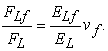(10)

Since the fiber stiffness is several times greater than the matrix stiffness, the second term in (7) may be neglected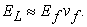(11)

### 4. Efective Transferse Modulus of Elasticity

In the following Figure we can see transverse load composite. The stress resultant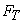respectively the stress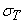is equal for all phase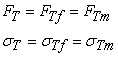(12)Download asVeiw figureFigures index
Figurr 2. Mechanical model to calculate the effective transverse modulus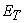From Figure 2 it follows that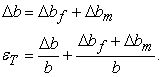(13)

end with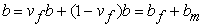(14)

and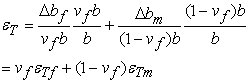(15)

with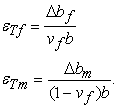(16)

Using Hooke´s law for the fiber, the matrix and the composite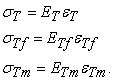(17)

Substituting Eqs. (17) in (16) and considering (5) gives to formula of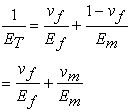(18)

or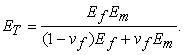(19)

Equation (19) is referred to Reuss estimate. The predicted value ofare seldom in good agreement with experimental results .Download asVeiw figureFigures index
Figure 3. Mechanical model to calculate the major Poisson´s ratio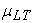### 5. Longitudial Moduls of Elasticity

Assume a composite is loaded in the on-axis direction as show Figure 3. The major Poison´s ratio is defined as the negative of the ratio of the normal strain in the transverse direction to the normal strain in the longitudinal direction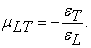(20)

With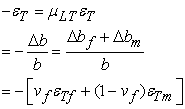(21)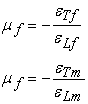(22)

it follows that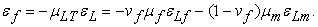(23)

The longitudinal strain in the composite, the fiber and the matrix are assumed to be equal and the equation for the major Poisson´s ratio reduces to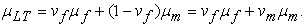(24)

The major Poisson´s ratioobeys the rule of mixture. The minor Poisson´s ratiocan be derived with the symmetry condition or reciprocal relationship.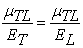(25)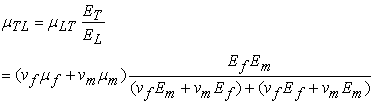(26)

The values of Poisson´s ratio for fiber or matrix rarely differ significantly, so that neither matrix nor fiber characteristic dominate the major or the minor elastic constantand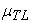.Download asVeiw figureFigures index
Figure 4. Mechanical model to calculate the effective in-plane shear modulus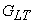### 6. Effective in – plane Sher Modulus

Apply a pure stress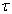to a lamina as shov in Figure 4. Assuming that the shear stresses on the fiber and the matrix are the same, but the shear strain are diferent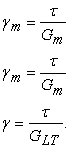(27)

The model is a connection in series and therefore(28)

and with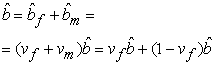(29)

it follows that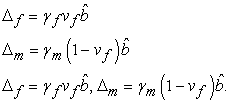(30)

Using Hooke´s law we get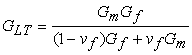(31)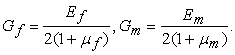(32)

### 7. Longitudial Moduls of Elasticity

Effective elastic moduli related to loading in the fiber direction, such asand, are dominated by the fibers. All estimations in this case and experimental results are very close to the rule of mixtures estimations. But the values obtained for transverse Young´s modulus and in-plane shear modulus with the rule of mixture which can be reduced to the two model connection of Voigt and Reuss, do not agree well with experimental results. This establishes a need for better modelling techniques based on elasticity solutions and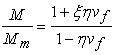(33)

variational principle models and includes analytical and solution methods.

Unfortunately, the theoretical models are only available in the form of complicated mathematical equations and the solution is very limited and needs huge effort. Semi-empirical relationship have been developed to overcome the difficult with purely theoretical approaches .

The most useful of those semi-empirical models are those of Halpin and Tsai which can be applied over a wide range of elastic properties and fiber volume fractions.

Starting from results obtained in theoretical analysis, Halpin and Tsai proposed equations that are general and simple in formulation. The moduli of a unidirectional composite are given by the following equationsandby the law of mixture Equation (9) and (20)

• For the other moduli by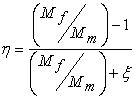(34)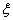- is called the reinforcement factor and depends on

• the geometry of the fibers

• the packing arrangement of the fibers

The more complicated formulas forandas the formulas given above by the rule of mixtures yields practically identical values to the simpler formulas and are not useful. But the elasticity solution for the modulusyields much better results and should be applied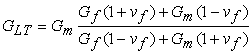(35)

The following recommendation may be possible for an estimate of effective elastic moduli of unidirectional laminaeandshould be estimate by the rule of mixturesfollows from the reciprocal conditionshould be (a) or the Halpin/Tsai formulas (34) and (33)may be estimate with help of the Halpin/Tsai formulas. But only when reliable experimental value ofandare available for a composite the factorcan be derivate for this case and can be used to predict effective moduli for a range of fiber volume ratio of the same composite .

It is also possible to look for numerical or analytical solutions forbased on elasticity theory. In generally,may vary from zero to infinity, and the Reuss and Voigt models are special case, e.g.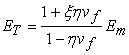(36)

for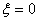and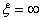, respectively .

### Acknowledgments

I would like to thank to Technical School in Kosice Department of applied mechanics and mechanical engineering. This research was supported by grants from the Slovak Grant Agency VEGA No. 1/0731/16.

### References

  R. F. Gibson, “Principles of Composite Material Mechanics,” Second Eds., McGraw –Hill, Inc. New York 1994.In article  H. Altenbach, J, Altenbach, W. Kissing, “Mechanics of Composite Structural Elements,” Springer-Verlag Berlin Heidelberg 2004.In article  L. P. Kollár and G. S. Springer, “Mechanicd of Composite Structures,” Cambridge University Press 2003.In article View Article  R. M. Jones, “Mechanics of Composite Materias,” Taylor and Francis, inc. 1999.In article  E. V. Morozov, “Mechanics and Analysis of Fabric Composites and Structures,” AUTEX Reseach Journal,Vol.4, No2, June 2004.In article